• matlab中的plot函数
万次阅读
2020-04-08 15:27:01

## 一．颜色

r红色
g绿色
b蓝色
c青色
m品红
y黄色
k黑色
w白色

使用方法：
plot（x，y，’color’，’颜色’）

## 二．形状

1. 连续点

-实线
–（这是两个线- -）虚线
虚线
-.点线

2. 离散点

离散点把离散点连起来
+-+
o（注意是英文o，不是数字0）-o（注意是英文o，不是数字0）
* （星型）-*
.（点型）-.
x或X-x或-X
square （正方形 ）-square
diamond （菱形）-diamond
pentagram （五角星）-pentagram
hexagram （六角星）-hexagram
^ （上三角）-^
v （下三角）-v
> （右三角）->
< （左三角）-<

使用方法：
plot（x，y，’形状’）matlab
更多相关内容
• 使用plot函数绘制图像 plot(x, y) 对图像属性进行设置，可以使用以下代码： p = plot(x, y); p.LineWidth = 2; %线宽为2 p.Color = 'r'; % 红色线条 p.Marker = '*' ; % 设置标记类型 p.MarkerSize = 6; %设置标记...

随机生成一组数据：

x = [1:1:10];
y = rand(1, 10);


使用plot函数绘制图像

plot(x, y)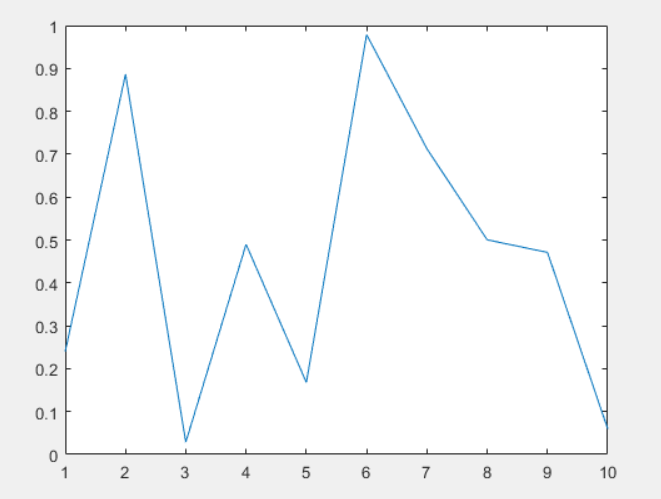对图像属性进行设置，可以使用以下代码：

p = plot(x, y);
p.LineWidth = 2; %线宽为2
p.Color = 'r';   % 红色线条
p.Marker = '*' ; % 设置标记类型
p.MarkerSize = 6; %设置标记大小
p.MarkerEdgeColor = 'b'; %设置标记边缘颜色
p.MarkerFaceColor = 'r'; %设置标记填充颜色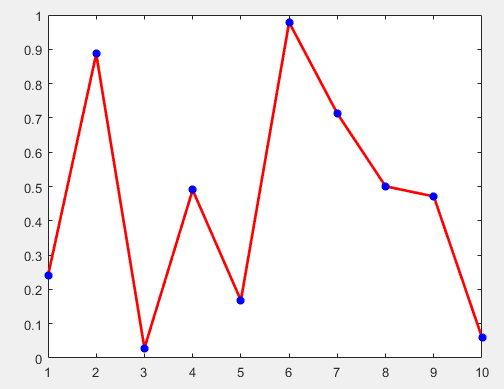set(gca, 'xtick', [3:1:12])  %自定义横坐标显示范围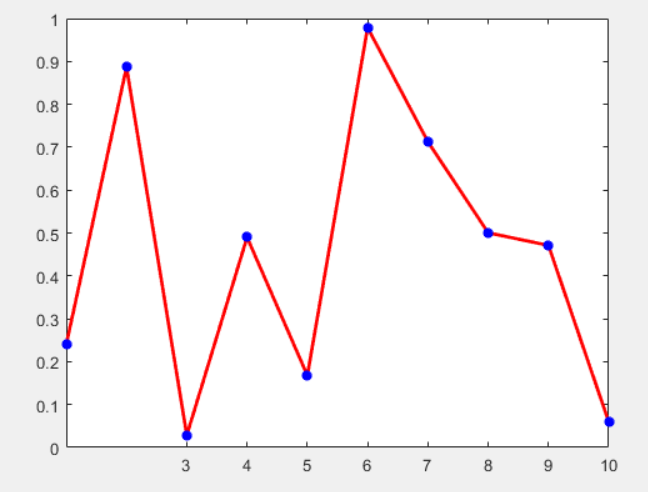set(gca, 'xticklabel', {'F1', 'F2', 'F3', 'F4', 'F5', 'F6', 'F7', 'F8', 'F9', 'F10' })  %自定义横坐标显示标签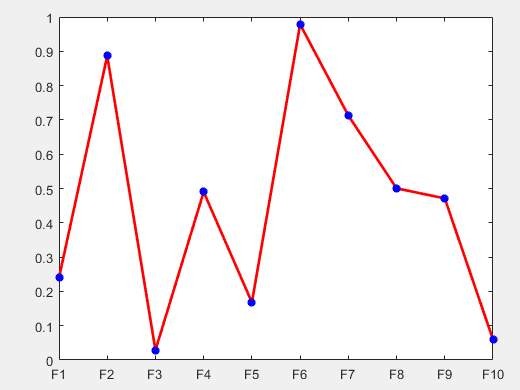添加图例

legend('Random Points')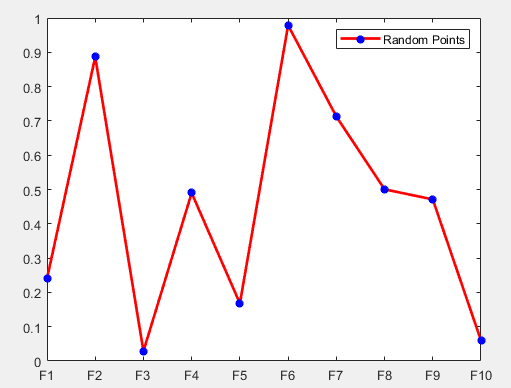设置坐标轴上下限：

axis([xmin,xmax,ymin,ymax]);


设置图片大小：

set(gcf,‘Position’,[x1,y1,dx,dy]);


x1和y1是图的左下角坐标， dx和dy是图沿着x和y方向的大小， 比如：

set(gcf,"Position",[347,162,380,300]);


图例的相关设置：
图例中字体及大小：

legend("FontName","Times New Roman","FontSize",7,LineWidth",1.5);


图例中各个量及位置：

legend("y","zc","location","SouthEast");


坐标轴的名称的相关设置：

x轴的名称及字体和大小：

xlabel("x(m)","FontName","Times New Roman","FontSize",7);


y轴的名称及字体和大小：

ylabel("zc(m) and y(m)","FontName","Times New Roman","FontSize",7,LineWidth",1.5);


坐标轴的字体及大小，以及坐标轴的刻度间隔

set(gca,'xtick', [1:1:10], "FontName","Times New Roman","FontSize",7,"LineWidth",1.5);


图名的字体及大小设置

title("yslice:600m;zslice:150m","FontName","Times New Roman","FontSize",7);


坐标轴反向

set(gca,"zdir","reverse"); %将坐标轴数值反向

展开全文matlab 开发语言
• 详细的介绍了MATlab中plot函数的用法，非常仔细，全面MATLAB
• ## MATLAB中plot函数的用法

万次阅读 多人点赞 2018-04-23 15:47:04
使用plot绘制二维图像本文转自http://blog.sina.com.cn/s/blog_d8f783c90102woqb.html以及https://blog.csdn.net/alvern_zhang/article/details/51153058MATLAB中plot函数常常被用于绘制各种二维图像，其用法也是...

## 使用plot绘制二维图像

本文转自http://blog.sina.com.cn/s/blog_d8f783c90102woqb.html

以及https://blog.csdn.net/alvern_zhang/article/details/51153058

MATLABplot函数常常被用于绘制各种二维图像，用法也是多种多样，本文仅介绍plot函数的基本用法——使用plot函数绘制二维点图和线图。plot函数的一般调用形式如下：

plot(X, Y, LineSpec)

其中X由所有输入点坐标的x值组成，Y是由与X中包含的x对应的y所组成的向量。LineSpec是用户指定的绘图样式，主要选项如下：

 Specifier Line Style（线型） --- 实线（默认样式）虚线（短划线） : 点线 -. 点划线

 Specifier Marker（坐标点样式） o 圆 + 加号 * 星号 . 点 x 十字 s 正方形 d 菱形 ^ 上指向三角形 v 下指向三角形 > 右指向三角形 < 左指向三角形 p 五角星 h 六角形

 Specifier Color y 黄色 m 品红 c 蓝绿色 r 红色 g 绿色 b 蓝色 w 白色 k 黑色

例如：’--or’表示坐标点为圆圈标志，且线型为红色短划线的绘图样式。

### 注意

在同时绘制多条曲线时，如果没有指定曲线属性，plot按顺序循环使用当前坐标系中ColorOrder和LineStyleOrder两个属性。

默认情况，MATLAB在每次调用plot函数时将ColorOrder和LineStyleOrder自动重置为DefaultAxesColorOrder和DefaultAxesLineStyleOrder。Default**属性我们可以自定义，有效期至MATLAB关闭，Matlab下次启动时将Default**属性重置为厂家设置(Factory)

set(0,’DefaultAxesColorOrder’,’r|g|b|k’,…’DefaultAxesLineStyleOrder’,’-|-.|–|:’)

使用hold all命令可以阻止调用plot函数时自动重置ColorOrder和LineStyleOrder属性，而是循环使用。注意hold on只是使多次绘制的图形叠加（相当于NextPlot），但不能阻止属性重置。

另外我们可以通过下面四个属性设置标识符的颜色和大小

LineWidth——指定线宽

MarkerEdgeColor——指定标识符的边缘颜色

MarkerFaceColor——指定标识符填充颜色

MarkerSize——指定标识符的大小

注意上面四个属性是针对当前坐标系中所有曲线的

### 实例

X=1:10;
% 两个都是数组，必须具有相同的尺寸
X1=[X;X;X]’;%10×3
Y1=rand(10,3)+1;%10×3% 其中一个为向量，另一个为数组，自动匹配尺寸相等方向
X2=1:0.1:10;%1×91
Y2=[sin(X2);cos(X2)]’;%91×2% 其中一个是标量，另一为矢量，绘制垂直坐标轴的离散点
X3=1:10;
Y3=-0.5;
fh=figure(‘numbertitle’,’off’,’name’,’PLOT Usability Demo’);%创建figure对象
ah=axes;%创建axes对象
h=plot(…%返回所有曲线句柄
ah,…%指定坐标系，可以省略，此时默认gca
X1,Y1,…%坐标数据
‘-.^’,…%曲线属性，可以省略或部分省略，此时自动选择
X2,Y2,…
‘m-‘,…
X3,Y3,…
‘o’,…%注意此组数据设置线型和颜色无效，因为默认绘制离散点
‘LineWidth’,2,…%线宽
‘MarkerEdgeColor’,’k’,…%标识符边缘颜色
‘MarkerFaceColor’,’r’,…%标识符填充颜色
‘MarkerSize’,8)%标识符大小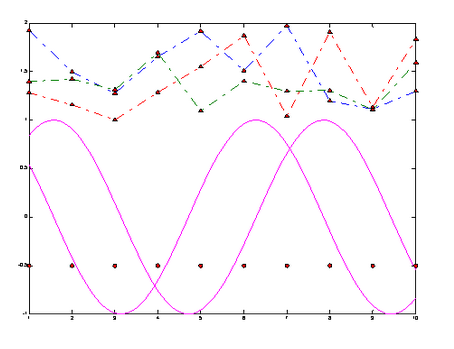举例如下：

定义x02pi之间的一组向量，且x向量中相邻两个值之间的增量为pi/100。定义y向量中的值为x向量的sine值。

x = 0:pi/100:2*pi;

y = sin(x);

figure 打开新的绘画窗口，可省略该句

plot(x,y) %采用默认样式，绘制实线

x = 0:pi/20:2*pi;

y = sin(x);

plot(x,y,'--or') %坐标点为圆圈标志，且线型为红色短划线的绘图样式

注：可以根据上述LineSpec表格自由组合线型样式。

在不关闭绘图窗口的前提下，还可以为图像添加标题，x轴和y轴标签，例如：

xlabel('x')

ylabel('sin(x)')

title('Plot of the Sine Function')

想要在现有的图片上添加另外一条线，可以使用hold语句，例如：

x = 0:pi/100:2*pi;

y = sin(x);

plot(x,y)

hold on

y2 = cos(x);

plot(x,y2,'r:')

legend('sin','cos') %为图片添加图例## 使用plot3绘制三维图像

MATLAB中有许多函数可以用来绘制三维图像，在此仅对plot3函数进行简要介绍，有兴趣的同学可以自行学习。plot3函数的调用形式如下：

plot3(X, Y, Z,LineSpec)

例如：

z=0:pi/50:10*pi;

x=sin(z);

y=cos(z);

plot3(x,y,z)

展开全文matlab
• ## matlab中plot函数用法

万次阅读 多人点赞 2019-03-06 14:41:15
1.简单的2维直线图 : plot(x,y) 同一坐标显示n条线：plot(x,y1,x,y2，…) x = 0:pi/10:2*pi; y = sin(x); figure; hold on; plot(x,y) 2.plot(X):X是矩阵，表示矩阵的每一行都画一条线，将显示n条线。 X=rand(3,3)...

线条、颜色等参数：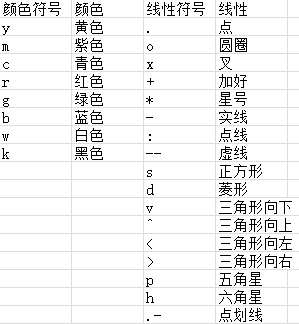1.简单的2维直线图 : plot(x,y)
同一坐标显示n条线：plot(x,y1,x,y2，…)

x = 0:pi/10:2*pi;
y = sin(x);
figure;
hold on;
plot(x,y)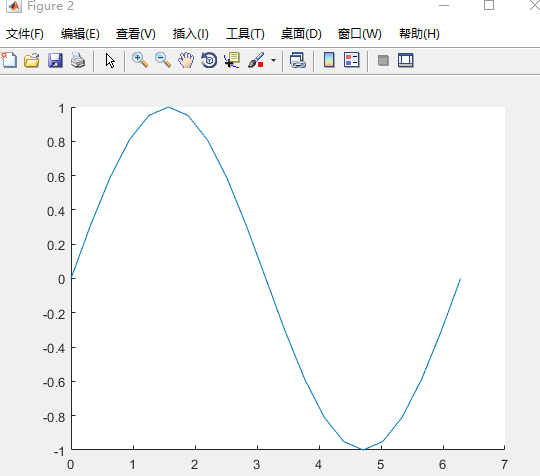2.plot(X):X是矩阵，表示矩阵的每一行都画一条线，将显示n条线。

X=rand(3,3); %随机生成3*3矩阵
figure;
hold on;
plot(X)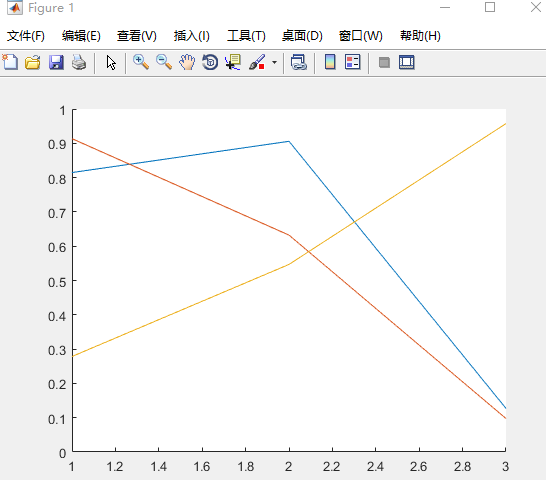3.画带形状的图

plot(X(pos, 1), X(pos, 2), 'k+','LineWidth', 2, 'MarkerSize', 7);
plot(X(neg, 1), X(neg, 2), 'ko', 'MarkerFaceColor', 'y','MarkerSize', 7);

X(pos, 1), X(pos, 2)表示横纵坐标
'k+'表示线性为加号型、黑色
LineWidth', 2表示线宽为2
'MarkerSize', 7表示形状大小为7
'MarkerFaceColor', 'y'表示形状填充颜色为黄色
另：MarkerEdgeColor','b'表示方框边缘颜色为蓝色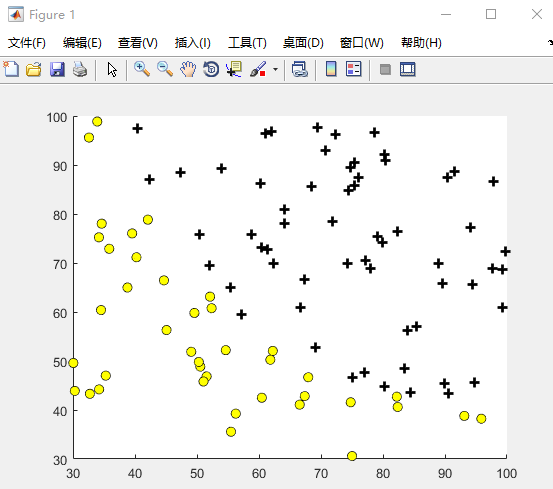展开全文matlab
• 使用matlab中plot函数需要用到的相关语句
• matlab plot函数详解 原创 ...matlab
• Matlab中plot函数及legend函数详解及实例.pdf
• Matlab中plot函数及legend函数详解及实例[参照].pdf
• ## MATLAB：plot函数详解

万次阅读 多人点赞 2021-08-08 20:50:29
本文介绍了MATLAB中plot函数的用法，方便日后适用matlab
• ## Matlab中plot函数及legend函数详解

千次阅读 多人点赞 2018-06-23 13:30:24
Matlab中plot函数及legend函数详解Matlab中plot函数及legend函数详解Matlab中plot函数全功能解析功能二维曲线绘图语法plot(Y)plot(X1,Y1,...)plot(X1,Y1,LineSpec,...)plot(...,'PropertyName',PropertyValue,...)...
• 转自 ... matlabplot函数画线时有很多的颜色和类型可以选择，下面列举一些。 常用的3+4+1=8种颜色：（速记：RGB、CYMK、W，即两种色彩空间模型+白色） '...
• plot(x,error,'ob','linewidth',LineWidth); % % 绘图标题，数轴标注，图例 title('M','fontname',FontName,'fontsize',TitleFontSize); xlabel('DetecedData(pixel)','fontname',FontName,'fontsize',...MATLAB
• 前段时间用SVR调参，需要对C的权重进行修改，所以上网搜...plt.plot(X, y, 'bo', fillstyle='none') plt.plot(X, result1, 'r.') 红色 plt.plot(X, result2, 'g.') 绿色 plt.plot(X, result3, 'c.') 亮蓝 plt.plot(...
• 功能 二维曲线绘图   语法 1 2 3 4 5 ...plot(Y) ...plot(X1,Y1,...) ...plot(X1,Y1,LineSpec,...) ...plot(...,'PropertyName',PropertyValue,...) ...plot(axes_handle
• 文章目录前言一、plot()函数二、代码运行结果总结 前言 此为本人学习的一些笔记总结，仅供参考。 提示：以下是本篇文章正文内容，下面案例可供参考 一、plot()函数 提示：关于plot()函数的部分叙述 1.plot(X,Y) ...
•matlab matlab 开发语言
• matlab中plot函数的坐标尺度一般是自动生成的，但是有时候是不太令人满意的，可根据以下方法修改尺度。 可通过此句代码实现，将这句代码放在plot函数之后即可。 set(gca,'xtick',0:1:20) 按照自己需求修改参数0:1:...matlab
• plot函数绘制二维的曲线 plot函数的原型 plot(X, Y, LineSpec) 其中X，Y是x轴的数值和对应的y的数值组成的向量 LineSpec是图像的绘制项式，其主要的参数选项如下表 比如 x=0:0.1:1 y=[-0.447,1.978,3.28,6.16,7.08...matlab
• ## MATLAB中plot函数功能详解

万次阅读 多人点赞 2017-06-18 21:34:11
基本功能 功能：二维曲线绘图 ...plot(Y) plot(X1,Y1,...) plot(X1,Y1,LineSpec,...) plot(...,'PropertyName',PropertyValue,...) plot(axes_handle,...) h = plot(...) hlines = plot('v6',...)   描述：matlab
• plot函数 功能 二维曲线绘图 语法 plot(Y) plot(X1,Y1,...) plot(X1,Y1,LineSpec,...) plot(...,'PropertyName',PropertyValue,...) plot(axes_handle,...) h = plot(...) 描述 plot(Y)如果Y是m×n的数组，以1...MATLAB
• 假设我的图像如下，我想用plot函数标记几个点，代码如下： plot(4,8,'ro','MarkerSize',5); plot(6,1,'ro','MarkerSize',5); 得到的结果图放大局部后，如下： 可以看到，输入的两个坐标分别为（4，8）、（6，1）...matlab
• ## Matlab的中Plot函数的基本用法(详解)

万次阅读 多人点赞 2019-05-14 15:23:02
本文接下来要讲解一下Matlab中最基本的Plot的用法 Plot的定义和用法 此 MATLAB 函数 创建 Y 数据对 X 对应值的二维线图。 如果 X 和 Y 都是向量，则它们的长度必须相同。plot 函数绘制 Y 对 X 的图。 如果 X ...Matlab
• x,y均为长度相同的向量 x(1:k)表示取x的前k个数 此图k = 20 plot(x,y,'bo',x(1:k),y(1:k),'g*') 效果图如下：matlab
• 在使用MATLABplot函数时，想在实现上加实点，刚开始使用的代码如下： figure;plot(rand(1,6),'-.') “-”表示实线，“.”表示实心点，但得到的却是点划线。 找了很久都没有找到答案，一番艰辛后，将代码的“.matlab
• plot(t,sin(t),'--mo','LineWidth',2,'MarkerEdgeColor','k','MarkerFaceColor',[.49 1 .63],'MarkerSize',12); %'--mo',这里是设置线型（--）和颜色（m），标记点类型(o，没有变默认为连续），后面一次是线的宽度...matlab...

# matlab中的plot函数matlab 订阅